## Example Questions

### Example Question #1 : How To Find The Area Of An Equilateral Triangle

What is the area of an equilateral triangle with a base of?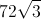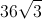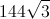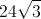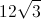Explanation:

An equilateral triangle can be considered to be 2 identical 30-60-90 triangles, giving the triangle a height of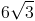. From there, use the formula for the area of a triangle: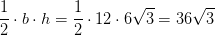### Example Question #1 : Equilateral Triangles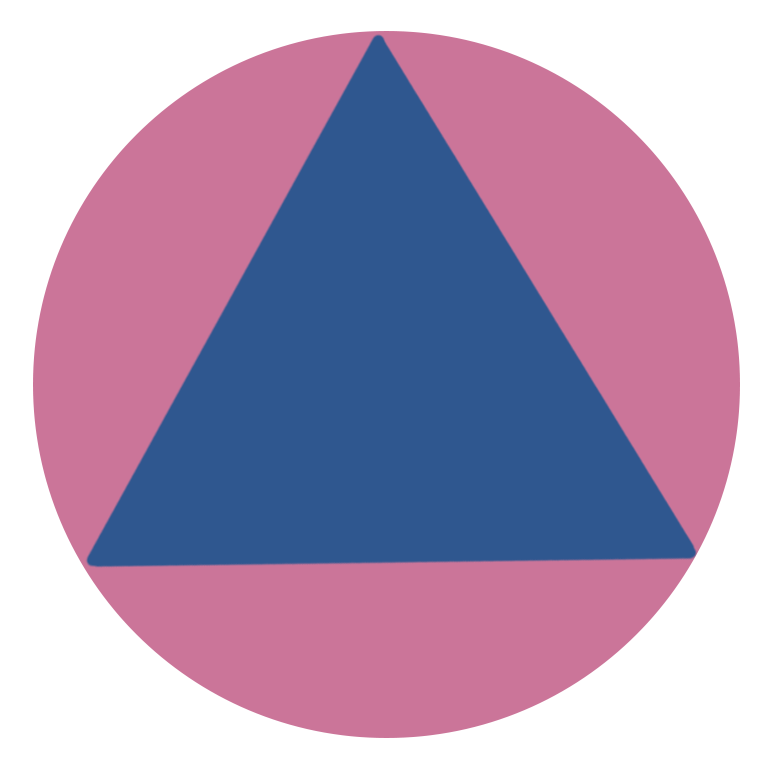An equilateral triangle is inscribed into a circle of radius 10. What is the area of the triangle?

The answer cannot be determined from the information given.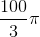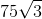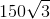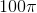Explanation:

To solve this equation, first note that a line drawn from the origin to a vertex of the equilateral triangle will bisect the angle of the vertex. Furthermore, the length of this line is equal to the radius: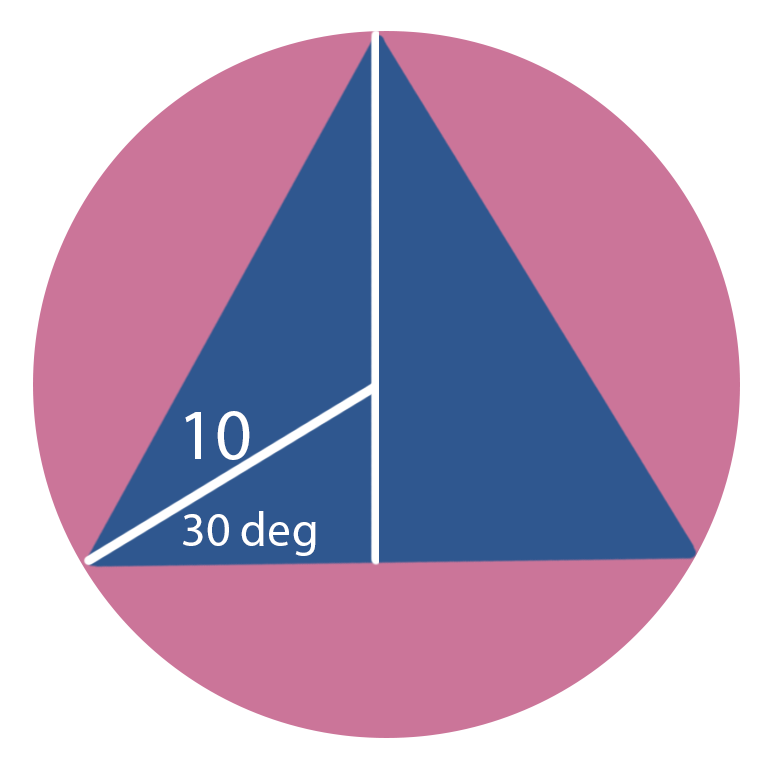That this creates in turn is a 30-60-90 right triangle. Recall that the ratio of the sides of a 30-60-90 triangle is given as: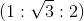Therefore, the length of theside can be found to be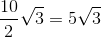This is also one half of the base of the triangle, so the base of the triangle can be found to be: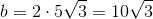Furthermore, the length of theside is: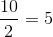The vertical section rising from the origin is the length of the radius, which when combined with the shorter section above gives the height of the triangle: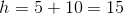The area of a triangle is given by one half the base times the height, so we can find the answer as follows: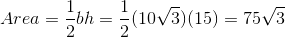### Example Question #33 : Triangles

Find the area of an equilateral triangle when one of its sides equals 4.

4

8

4√3

2√3

4√3

Explanation:

All sides of an equilateral triangle are equal, so all sides of this triangle equal 4.

Area = 1/2 base * height, so we need to calculate the height: this is easy for an equilateral triangle, since you can bisect any such triangle into two identical 30:60:90 triangles.

The ratio of lengths of a 30:60:90 triangle is 1:√3:2. The side of the equilateral triangle is 4, and we divided the base in half when we bisected the triangle, so that give us a length of 2, so our triangle must have sides of 2, 4, and 2√3; thus we have our height.

One of our 30:60:90 triangles will have a base of 2 and a height of 2√3. Half the base is 1, so 1 * 2√3 = 2√3.

We have two of these triangles, since we divided the original triangle, so the total area is 2 * 2√3 = 4√3.

You can also solve for the area of any equilateral triangle by applying the formula (s2√3)/4, where s = the length of any side.

Tired of practice problems?

Try live online GRE prep today.